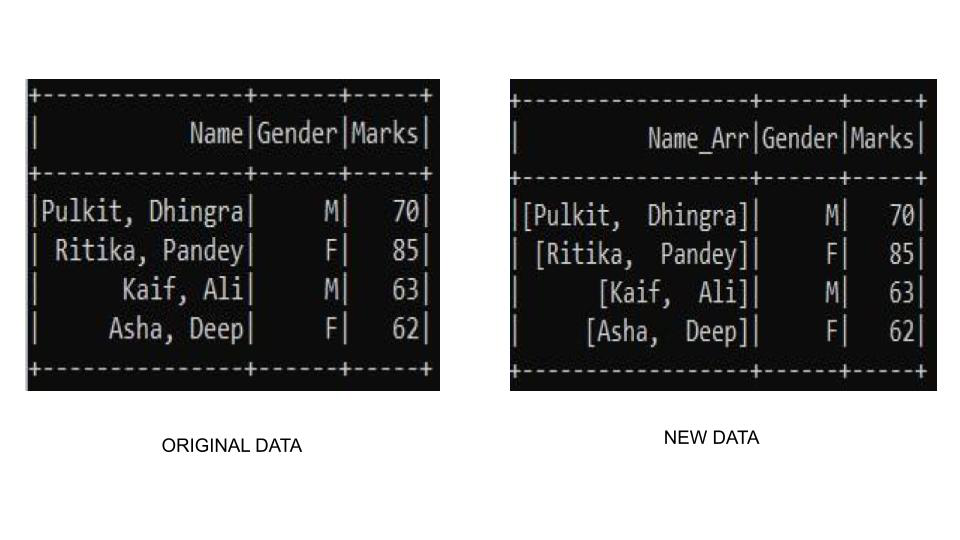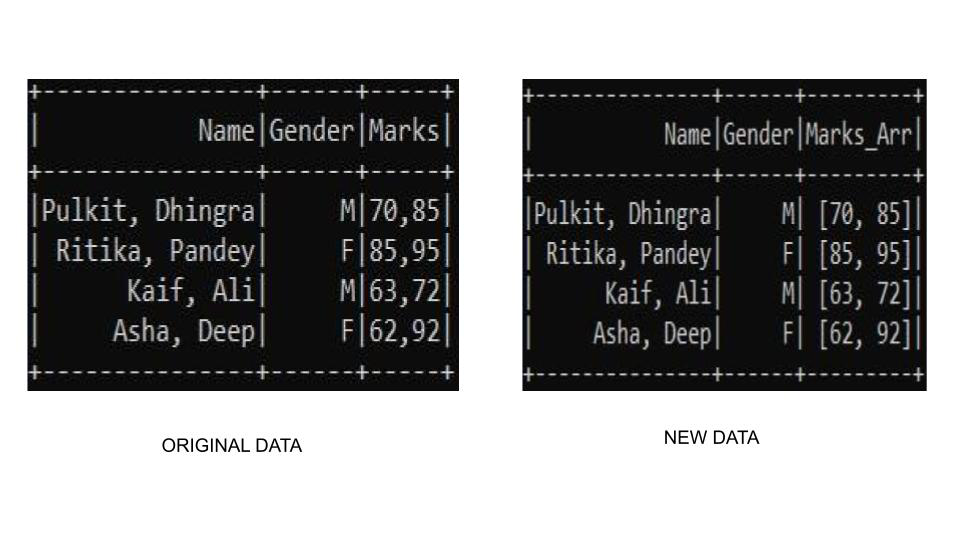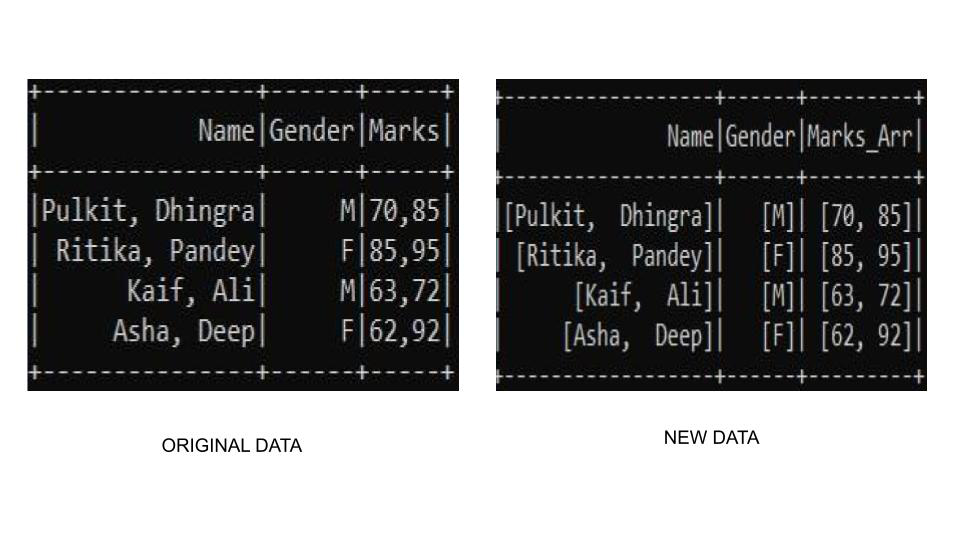# Convert comma separated string to array in PySpark dataframe

• Last Updated : 23 May, 2021

In this article, we will learn how to convert comma-separated string to array in pyspark dataframe.

In pyspark SQL, the split() function converts the delimiter separated String to an Array.  It is done by splitting the string based on delimiters like spaces, commas, and stack them into an array. This function returns pyspark.sql.Column of type Array.

Attention geek! Strengthen your foundations with the Python Programming Foundation Course and learn the basics.

To begin with, your interview preparations Enhance your Data Structures concepts with the Python DS Course. And to begin with your Machine Learning Journey, join the Machine Learning - Basic Level Course

Syntax: pyspark.sql.functions.split(str, pattern, limit=-1)

Parameter:

• str:- The string to be split.
• limit:- an integer that controls the number of times pattern is applied
• pattern:- The delimiter that is used to split the string.

### Examples

Let’s look at few examples to understand the working of the code.

Example 1: Working with String Values

Let’s look at a sample example to see the split function in action. For this example, we have created our custom dataframe and use the split function to create a name contacting the name of the student. Here we are going to apply split to the string data format columns.

## Python3

 `# import required modules``from` `pyspark.sql ``import` `SparkSession``from` `pyspark.sql.functions ``import` `split, col`` ` `# start the spark session``spark ``=` `SparkSession.builder \``         ``.appName(``'GeeksforGeeks'``) \``         ``.getOrCreate()`` ` `# create the dataframe``data ``=` `[(``"Pulkit, Dhingra"``,``"M"``,``70``),``            ``(``"Ritika, Pandey"``,``"F"``,``85``),``            ``(``"Kaif, Ali"``,``"M"``,``63``),``            ``(``"Asha, Deep"``,``"F"``,``62``)``            ``]`` ` `columns``=``[``"Name"``,``"Gender"``,``"Marks"``]``df``=``spark.createDataFrame(data,columns)`` ` `# use split function ``df2 ``=` `df.select(split(col(``"Name"``),``","``).alias(``"Name_Arr"``),``                ``col(``"Gender"``),col(``"Marks"``)) \``    ``.drop(``"Name"``)`` ` `df2.show()`` ` `# stop session``spark.stop()`

Output:Example 2: Working with Integer Values

If we want to convert to the numeric type we can use the cast() function with split() function. In this example we are using the cast() function to build an array of integers, so we will use cast(ArrayType(IntegerType())) where it clearly specifies that we need to cast to an array of integer type.

## Python3

 `# import required modules``from` `pyspark.sql ``import` `SparkSession``from` `pyspark.sql.functions ``import` `split, col``from` `pyspark.sql.types ``import` `ArrayType, IntegerType`` ` `# start the spark session``spark ``=` `SparkSession.builder \``         ``.appName(``'GeeksforGeeks'``) \``         ``.getOrCreate()`` ` `# create the dataframe``data ``=` `[(``"Pulkit, Dhingra"``,``"M"``,``"70,85"``),``            ``(``"Ritika, Pandey"``,``"F"``,``"85,95"``),``            ``(``"Kaif, Ali"``,``"M"``,``"63,72"``),``            ``(``"Asha, Deep"``,``"F"``,``"62,92"``)``            ``]`` ` `columns``=``[``"Name"``,``"Gender"``,``"Marks"``]``df``=``spark.createDataFrame(data,columns)``df.show()`` ` `# use split function ``df2 ``=` `df.select(col(``"Name"``),col(``"Gender"``),``split(col(``"Marks"``),``","``).cast(``  ``ArrayType(IntegerType())).alias(``"Marks_Arr"``))`` ` `df2.show()`` ` `# stop session``spark.stop()`

Output:Example 3: Working with both Integer and String Values

There may be a condition where we need to check for each column and do split if a comma-separated column value exists. The split() function comes loaded with advantages. There might a condition where the separator is not present in a column. The split() function handles this situation by creating a single array of the column value in place of giving an exception. This may come in handy sometimes.

## Python3

 `# If you want to convert data to numeric``# types you can cast as follows``import` `findspark``findspark.init(``'c:/spark'``)`` ` `# import required modules``from` `pyspark.sql ``import` `SparkSession``from` `pyspark.sql.functions ``import` `split, col``from` `pyspark.sql.types ``import` `ArrayType, IntegerType`` ` `def` `return_array(column):``        ``return` `split(col(column),``","``)`` ` `# start the spark session``spark ``=` `SparkSession.builder \``         ``.appName(``'GeeksforGeeks'``) \``         ``.getOrCreate()`` ` `# create the dataframe``data ``=` `[(``"Pulkit, Dhingra"``,``"M"``,``"70,85"``),``            ``(``"Ritika, Pandey"``,``"F"``,``"85,95"``),``            ``(``"Kaif, Ali"``,``"M"``,``"63,72"``),``            ``(``"Asha, Deep"``,``"F"``,``"62,92"``)``            ``]`` ` `columns``=``[``"Name"``,``"Gender"``,``"Marks"``]``df``=``spark.createDataFrame(data,columns)``df.show()`` ` `# use split function ``df2 ``=` `df.select(split(col(``"Name"``),``","``).alias(``"Name"``),``    ``split(col(``"Gender"``),``","``).alias(``"Gender"``),``    ``split(col(``"Marks"``),``","``).alias(``"Marks_Arr"``))``df2.show()`` ` `# stop session``spark.stop()`

Output:My Personal Notes arrow_drop_up